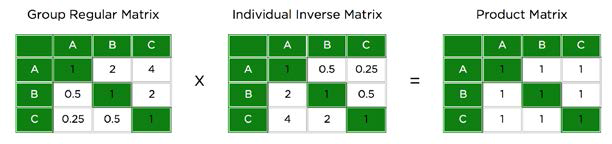# How is Alignment Index calculated?

The Alignment Index algorithm calculates the level of consensus there is around the judgments of a group. It provides a valuable heuristic to understand if individuals are generally on the same page (they valued objectives with similar priority), or if they were very different in their perceptions of relative importance of the objectives.

The alignment index shows how similar a group’s thinking is when looking at their final priorities. If individuals’ priorities differ widely from the overall group, the alignment score will be low; if everybody voted exactly the same, the alignment score would be as high as possible, in this case 100. To calculate the alignment index, we take each individual’s entire priority set and compare it to the group’s priority set by multiplying the transpose of the individual’s matrix (rows become columns, and vice versa) by the group’s matrix.Suppose that an individual and a group both voted 9 on the same comparison. The matrix of the individual’s transpose matrix with the group’s matrix would be (1/9)*9=1, because the individual’s matrix has been flipped on its diagonal. Therefore, if an individual’s judgment were in exact agreement with the group, the sum across each row would be (N*1)*N, or N^2. If any judgments are different, this will no longer be the case, and the total sum will be larger than N^2.

To see this, consider that the multiplied matrix will contain two references to the same comparison, one with each element as the row element. The sum of these two references is: The group alignment index is calculated by taking all of the ratios between each matrix’s row sum and N^2, averaging them, and then normalizing that to a 0 to 100 scale, where 100 is perfect alignment and 0 is what alignment would be if all voters were as far from the average as possible.